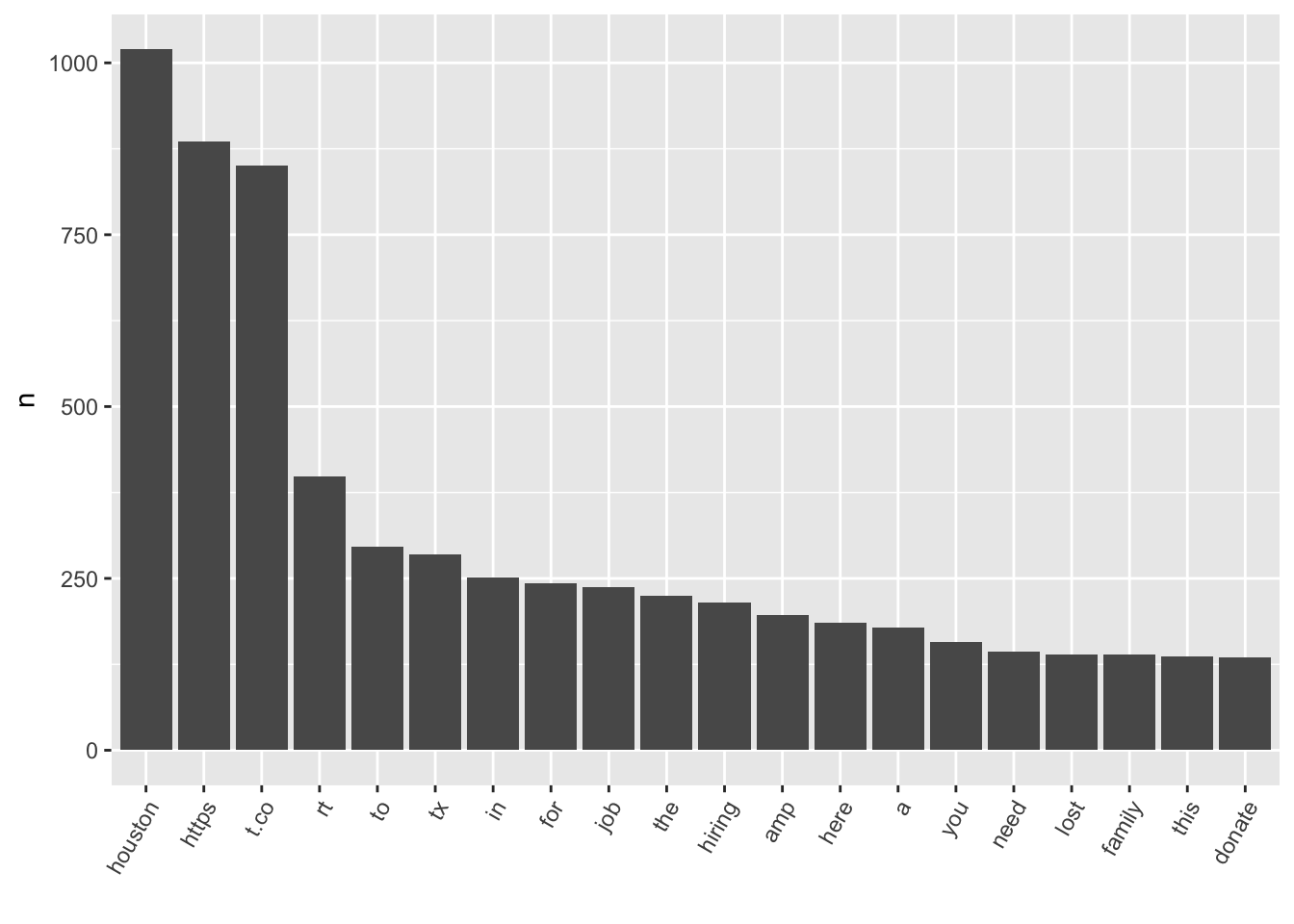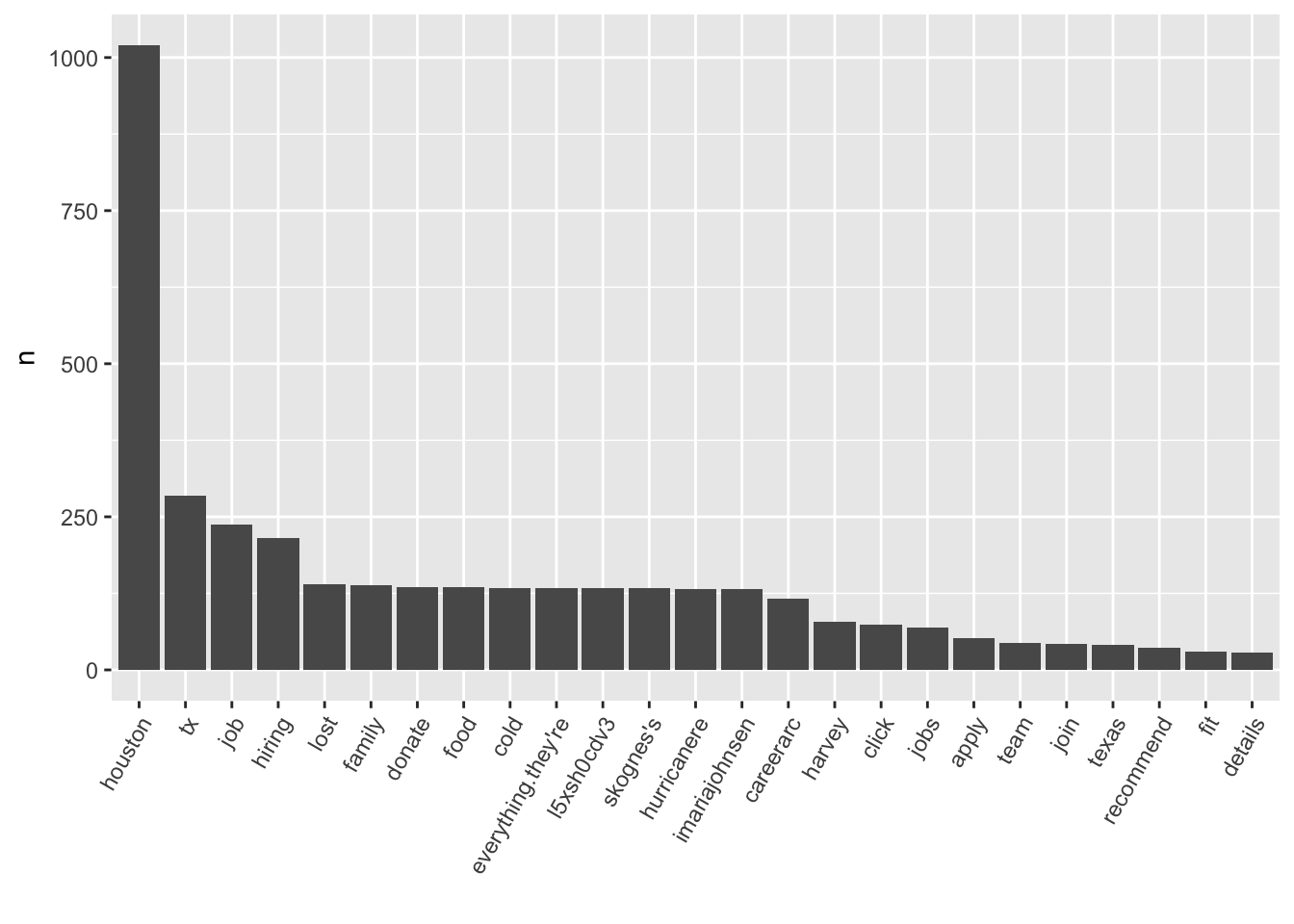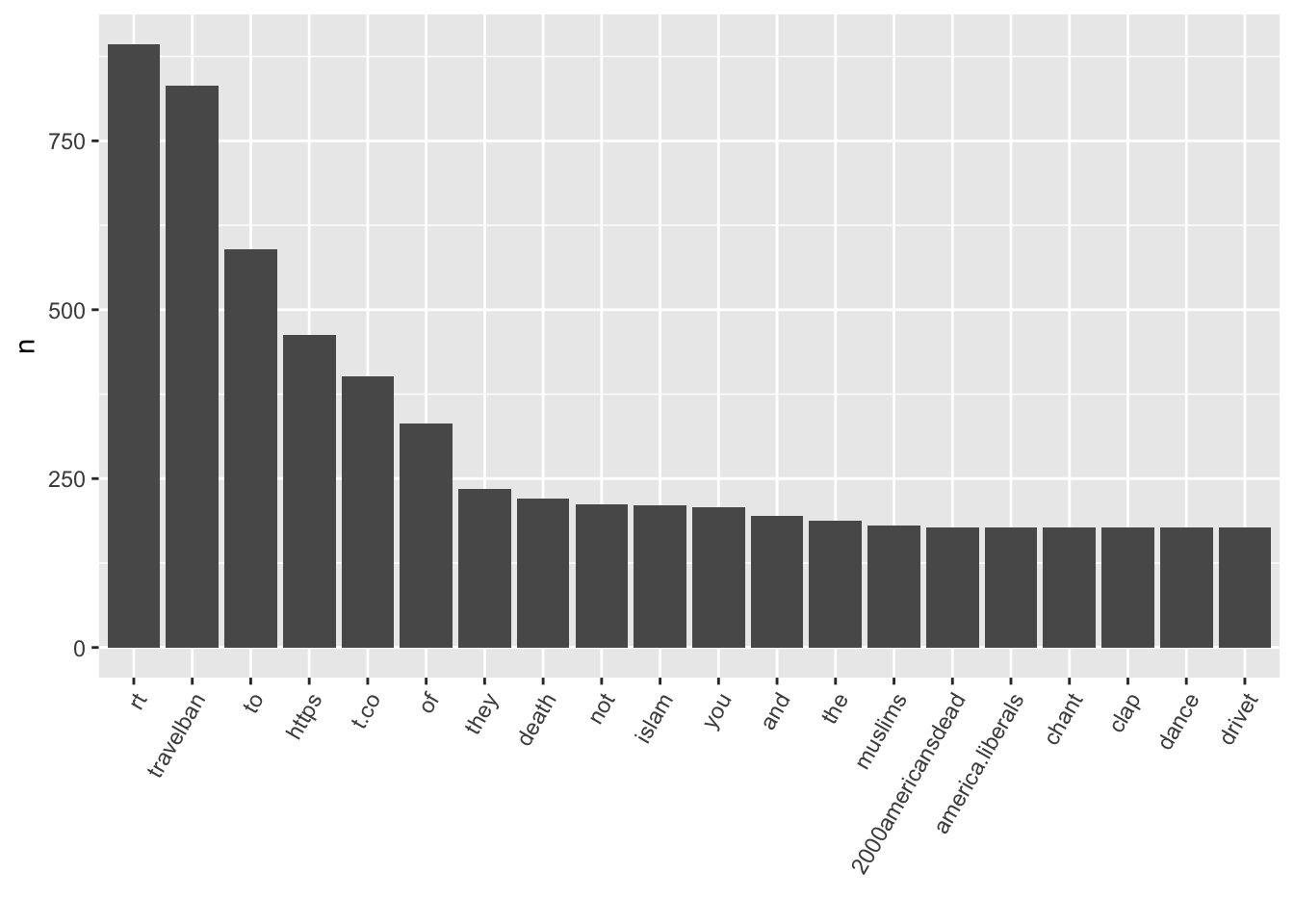This is based on:

1. Install the twitteR package and make it available in your R session.
``````#install.packages("twitteR")
#install.packages("tidytext")
#install.packages("dplyr")
#install.packages("ggplot2")``````
1. Now on the Twitter side you need to do a few things to get setup if you have not done them already.
• You need to have a twitter account. If you do not have one go to http://twitter.com/signup and set one up. Also you need to have a modile number as part of this account.

• Once you click on the “Create New App” button you will go to the Create an Application screen. There are three fields, a click box and a button you need to click on this page. The three fields are Name, Description and Website. The name of the application must be unique so this may take a few tries. The description needs to be at least 10 character long, and put in a website. If you do not have one you can use https://bigcomputing.blogspot.com. Now click the “Yes, I agree” box for the license agreement and click the “Create your Twitter application”.

``````library(twitteR)

consumer_key <- consumer_key_nt
consumer_secret <- consumer_secret_nt
access_token <- access_token_nt
access_secret <- access_secret_nt

``##  "Using direct authentication"``

# Example analyses

## Fake News

Now let’s search for fakenews, plot the frequent words within these tweets, and do a quick sentiment analysis.

``````library(tidyverse)

tweet_words <- fn_twitter_df %>% select(id, text) %>% unnest_tokens(word,text)

tweet_words %>% count(word,sort=T) %>% slice(1:20) %>%
ggplot(aes(x = reorder(word,
n, function(n) -n), y = n)) + geom_bar(stat = "identity") + theme(axis.text.x = element_text(angle = 60,
hjust = 1)) + xlab("")````````````# Create a list of stop words: a list of words that are not worth including

my_stop_words <- stop_words %>% select(-lexicon) %>%
bind_rows(data.frame(word = c("https", "t.co", "rt", "amp","4yig9gzh5t","fyy2ceydhi","78","fakenews")))

tweet_words_interesting <- tweet_words %>% anti_join(my_stop_words)

tweet_words_interesting %>% group_by(word) %>% tally(sort=TRUE) %>% slice(1:25) %>% ggplot(aes(x = reorder(word,
n, function(n) -n), y = n)) + geom_bar(stat = "identity") + theme(axis.text.x = element_text(angle = 60,
hjust = 1)) + xlab("")````````````bing_lex <- get_sentiments("nrc")

fn_sentiment <- tweet_words_interesting %>% left_join(bing_lex)

fn_sentiment %>% filter(!is.na(sentiment)) %>% group_by(sentiment) %>% summarise(n=n())``````
``````## # A tibble: 10 x 2
##       sentiment     n
##           <chr> <int>
##  1        anger   124
##  2 anticipation   245
##  3      disgust    80
##  4         fear   191
##  5          joy   315
##  6     negative   552
##  7     positive  1023
##  9     surprise   128
## 10        trust   569``````

## U.S. Travel Ban

Let’s do a similar analysis for travel ban tweets.

``````library(tidyverse)

tweet_words <- tb_twitter_df %>% select(id, text) %>% unnest_tokens(word,text)

tweet_words %>% count(word,sort=T) %>% slice(1:20) %>%
ggplot(aes(x = reorder(word,
n, function(n) -n), y = n)) + geom_bar(stat = "identity") + theme(axis.text.x = element_text(angle = 60,
hjust = 1)) + xlab("")````````````# Create a list of stop words: a list of words that are not worth including

my_stop_words <- stop_words %>% select(-lexicon) %>%
bind_rows(data.frame(word = c("https", "t.co", "rt", "amp","travelban")))

tweet_words_interesting <- tweet_words %>% anti_join(my_stop_words)

tweet_words_interesting %>% group_by(word) %>% tally(sort=TRUE) %>% slice(1:25) %>% ggplot(aes(x = reorder(word,
n, function(n) -n), y = n)) + geom_bar(stat = "identity") + theme(axis.text.x = element_text(angle = 60,
hjust = 1)) + xlab("")``````